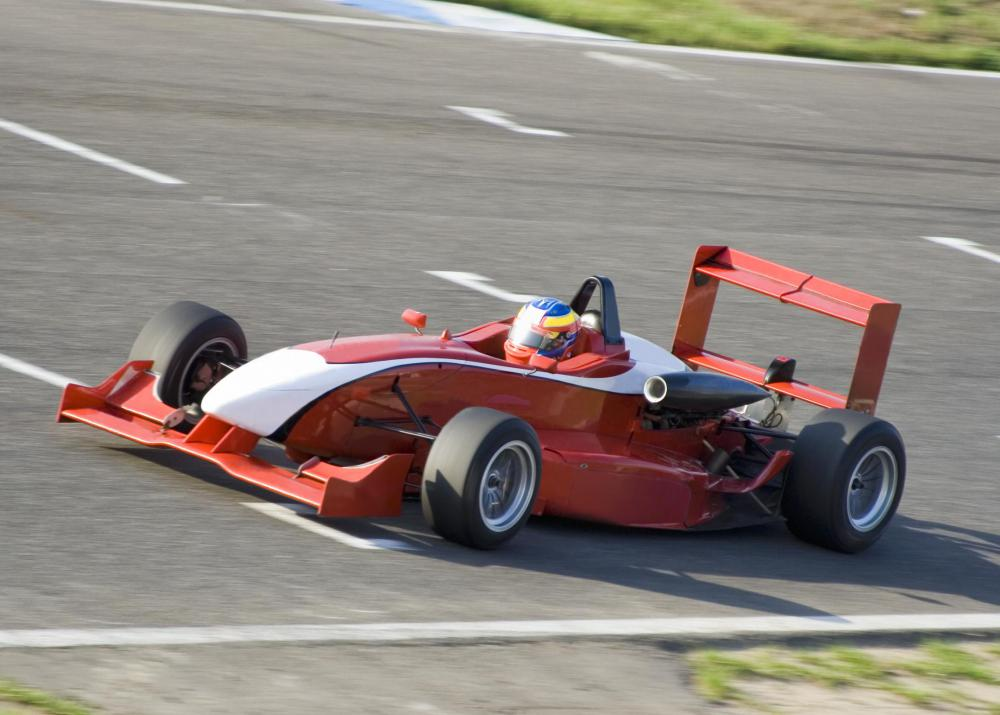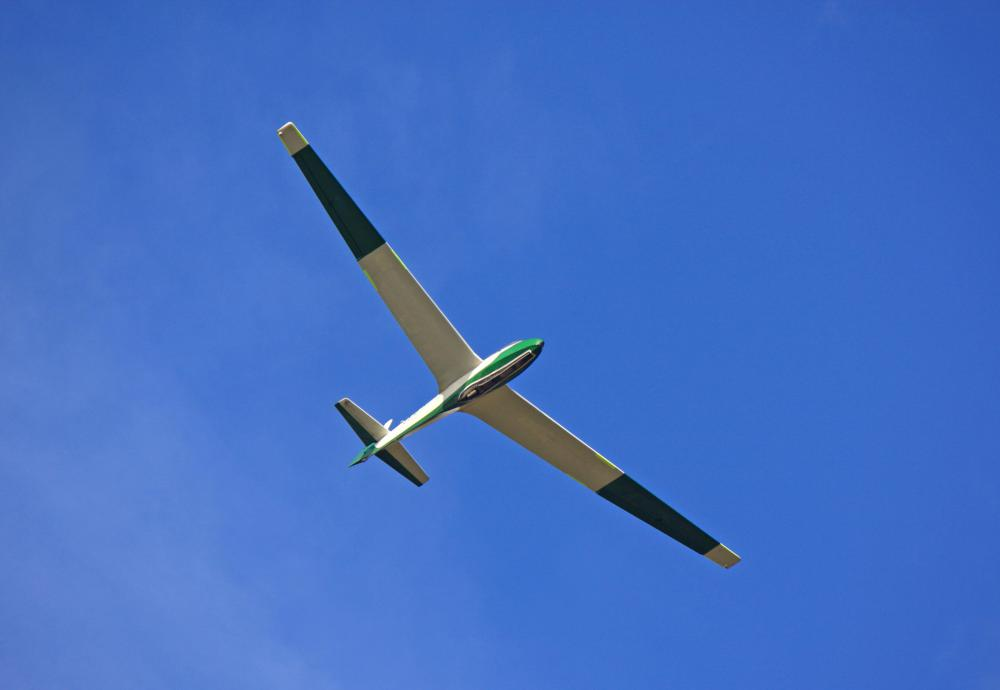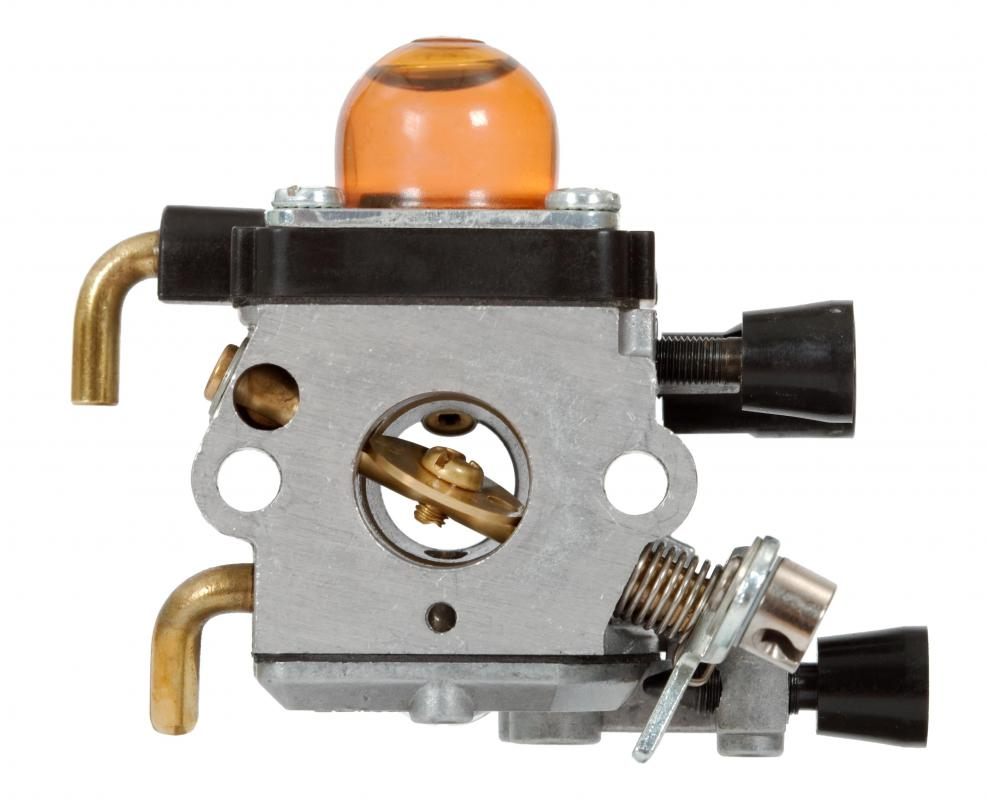# What is the Bernoulli Effect?

B. Nolta

The Bernoulli effect, or the Bernoulli principle or Bernoulli's law, is a statement of relationship between flow speed and pressure in a fluid system; in essence, when the speed of horizontal flow through a fluid increases, the pressure decreases. This effect, and the principle which states this formally, was discovered by the renowned mathematician Daniel Bernoulli, who first published its formulation in 1738. Since the word "fluid" in physics refers to the behavior of both liquids and gasses, such as air, the Bernoulli effect can be observed in both hydrodynamic, or fluid, systems as well as aerodynamic, or gaseous, systems.

A common example used to explain the Bernoulli effect is the flow of fluid through a pipe. If the fluid is moving uniformly through the pipe, then the only forces acting on the fluid are its own weight and the pressure of the fluid itself. Now, if the pipe narrows, the fluid must speed up, because the same amount of fluid is traveling through a smaller space. However, if the fluid is moving uniformly, and the weight has not changed, then the only way in which the fluid will move faster is if the pressure behind the fluid is greater than the pressure in front. Thus, the pressure must decrease as the speed increases.A racecar's spoilers and tailfins generate a downward force that helps it stay on track during tight turns.

Since air can be considered a fluid (in physics, anything that flows is considered a fluid), the Bernoulli effect is frequently used in modeling aerodynamic systems. Race cars use the Bernoulli effect to help keep them on the road at high speeds; by using a tail fin angled so that the air pressure above the fin is higher than below, the car is essentially held down by atmospheric pressure. This keeps the car on the road by negating "drift," and allows the driver greater control and safety. Airplanes use the Bernoulli effect in the opposite manner to help generate lift. Other common, real-world applications of the Bernoulli effect include carburetors, which apply the effect to draw in and mix air and fuel, and sailing ships, which can generate propulsive force from both the wind and the pressure differential described by Bernoulli’s principle.The wings of aircraft and gliders use the Bernoulli effect to generate lift.

Although there are different formulations of Bernoulli’s equation, which is a formal mathematical statement of the principle, the Bernoulli effect can be observed in each type of system that the formulations describe. Each formulation describes a different set of fluid characteristics — incompressible flow, unsteady potential flow and so on — but the Bernoulli effect is generally observable in each system. However, there are certain cases where the principle is invalid, and in those cases, the effect is as well.A common, real-world application of the Bernoulli effect is the carburetor, which applies the effect to draw in and mix air and fuel.

## You might also Likeanon991402

It is good information. It helped me a lot in science.

anon343257

There is more to the effect than stated!

anon319765

Thanks! This helped me understand how a HDD writes to the platters to store my data.

anon143225

is the same thing used for flutes?

anon87469

How are voltage and the Bernoulli Effect related?

anon79997

Now I understand completely. Thanks.

anon77065

really useful. thanks.

anon68469

thanks for the information. it really helps for my project.

anon50282

Thanks for the help!

anon36878

very useful information.